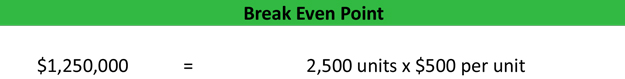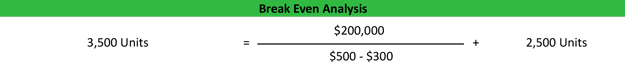## The Breakeven Point Formula can shine a light on whether your business can live or die.

That’s what the breakeven point equation is all about. Quite simply the life or death of a business.  When used effectively it the formula can help guide you and your business in turbulent times, helping your business reach the shore os business success.

We will help demystify the formula for you as best as we can, but if you still have any questions, please do not hesitate to drop your questions in the comment section below.

## The Breakeven Point

A company’s breakeven point is the point at which its sales exactly cover its expenses. To compute a company’s breakeven point in sales volume, you need to know the values of three variables:

• Fixed costs: Costs that are independent of sales volume, such as rent
• Variable costs: Costs that are dependent on sales volume, such as the cost of manufacturing the product
• The selling price of the product.

## How to Calculate Breakeven Point

In order to calculate your company’s breakeven point, use the following formula:

Fixed Costs ÷ (Price – Variable Costs) = Breakeven Point in Units

In other words, the breakeven point is equal to the total fixed costs divided by the difference between the unit price and variable costs. Note that in this formula, fixed costs are stated as a total of all overhead for the firm, whereas Price and Variable Costs are stated as per unit costs—​​the price for each product unit sold.

The denominator of the equation, price minus variable costs, is called the contribution margin. After unit variable costs are deducted from the price, whatever is left—​​​the contribution margin—​is available to pay the company’s fixed costs.

## An Example of Finding the Breakeven Point

XYZ Corporation has calculated that it has fixed costs that consist of its lease, depreciation of its assets, executive salaries, and property taxes. Those fixed costs add up to \$60,000. Their product is the widget. Their variable costs associated with producing the widget are raw material, factory labor, and sales commissions. Variable costs have been calculated to be \$0.80 per unit. The widget is priced at \$2.00 each.

Given this information, we can calculate the breakeven point for XYZ Corporation’s product, the widget, using our formula above:

\$60,000 ÷ (\$2.00 – \$0.80) = 50,000 units

What this answer means is that XYZ Corporation has to produce and sell 50,000 widgets in order to cover their total expenses, fixed and variable. At this level of sales, they will make no profit but will just break even.

## What Happens to the Breakeven Point If Sales Change

What if your sales change? For example, if the economy is in a recession, your sales might drop. If sales drop, then you may risk not selling enough to meet your breakeven point. In the example of XYZ Corporation, you might not sell the 50,000 units necessary to break even.

In that case, you would not be able to pay all your expenses. What can you do in this situation? If you look at the breakeven formula, you can see that there are two solutions to this problem: you can either raise the price of your product or you can find ways to cut your costs, both fixed and variable.

## How Cutting Costs Affects the Breakeven Point

Let’s say you find a way to cut the cost of your overhead or fixed costs by reducing your own salary by \$10,000. That makes your fixed costs drop from \$60,000 to \$50,000. Using the same formula and holding all other variables the same, the breakeven point would be:

\$50,000 ÷ (\$2.00-\$0.80) = 41,666 units

Predictably, cutting your fixed costs drops your breakeven point. If you reduce your variable costs by cutting your costs of goods sold to \$0.60 per unit, on the other hand, then your breakeven point, holding other variables the same, becomes:

\$60,000 ÷ (\$2.00-\$0.60) = 42,857 units

From this analysis, you can see that if you can reduce the cost variables, you can lower your breakeven point without having to raise your price.

## Relationships Between Fixed Costs, Variable Costs, Price, and Volume

As the owner of a small business, you can see that any decision you make about pricing your product, the costs you incur in your business, and sales volume are interrelated. Calculating the breakeven point is just one component of cost-volume-profit analysis, but it’s often an essential first step in establishing a sales price-point that ensures a profit.

## Example

Let’s take a look at an example of each of these formulas. Barbara is the managerial accountant in charge of a large furniture factory’s production lines and supply chains. She isn’t sure the current year’s couch models are going to turn a profit and what to measure the number of units they will have to produce and sell in order to cover their expenses and make at \$500,000 in profit. Here are the production stats.

• Total fixed costs: \$500,000
• Variable costs per unit: \$300
• Sale price per unit: \$500
• Desired profits: \$200,000

First we need to calculate the break-even point per unit, so we will divide the \$500,000 of fixed costs by the \$200 contribution margin per unit (\$500 – \$300).As you can see, the Barbara’s factory will have to sell at least 2,500 units in order to cover it’s fixed and variable costs. Anything it sells after the 2,500 mark will go straight to the CM since the fixed costs are already covered.

Next, Barbara can translate the number of units into total sales dollars by multiplying the 2,500 units by the total sales price for each unit of \$500.Now Barbara can go back to the board and say that the company must sell at least 2,500 units or the equivalent of \$1,250,000 in sales before any profits are realized. She can also take it a step further and use a break-even point calculator to compute the total number of units that must be produced in order to meet her \$200,000 profitability goal by dividing the \$200,000 desired profit by the contribution margin then adding the total number of break-even point units.These are just examples of the break-even point. You can use these as a template for your business or course work.

To get a better sense of what this all means, let’s take a more detailed look at the formula components.

• Fixed costs: As noted above, fixed costs are not affected by the number of items sold, such as rent paid for storefronts or production facilities, computers, and software. Fixed costs also include fees paid for services like graphic design, advertising, and public relations.
• Contribution margin: The contribution margin is calculated by subtracting an item’s variable costs from the selling price. So if you’re selling a product for \$100 and the cost of materials and labor is \$40, then the contribution margin is \$60. This \$60 is then used to cover the fixed costs, and if there is any money left after that, it’s your net profit.
• Contribution margin ratio: This figure, usually expressed as a percentage, is calculated by subtracting your fixed costs from your contribution margin. From there, you can determine what you need to do to break even, like cutting production costs or raising your prices.
• Profit earned following your break even: Once your sales equal your fixed and variable costs, you have reached the break-even point, and the company will report a net profit or loss of \$0. Any sales beyond that point contribute to your net profit.